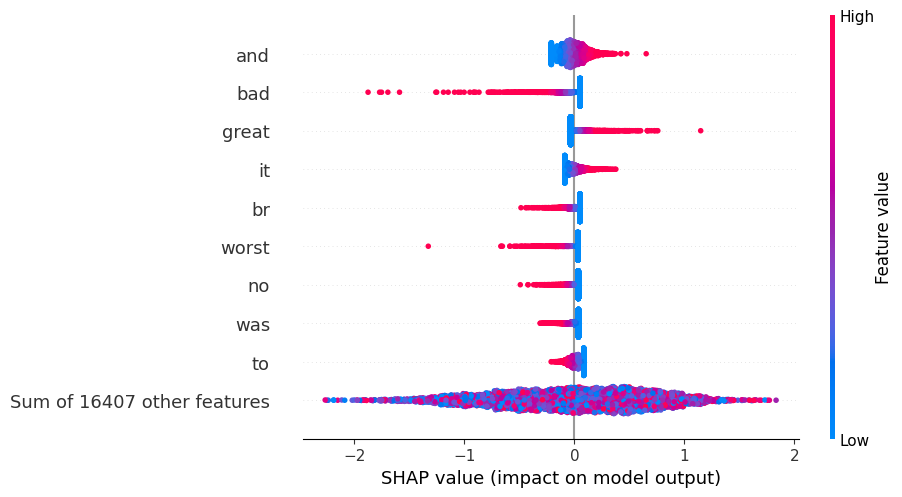# Sentiment Analysis with Logistic Regression

This gives a simple example of explaining a linear logistic regression sentiment analysis model using shap. Note that with a linear model, the SHAP value of feature $$i$$ for the prediction $$f(x)$$ (assuming feature independence) is just $$\phi_i = \beta_i \cdot (x_i - E[x_i])$$. Since we are explaining a logistic regression model, the units of the SHAP values will be in the log-odds space.

The dataset we are using is the classic IMDB dataset from this paper. When explaining the model, it is interesting to observe how the words that are absent from the text are sometimes just as important as those that are present.

:

import sklearn
from sklearn.feature_extraction.text import TfidfVectorizer
from sklearn.model_selection import train_test_split
from sklearn.metrics import classification_report
import numpy as np
import shap
np.random.seed(101)
shap.initjs():

corpus,y = shap.datasets.imdb()
corpus_train, corpus_test, y_train, y_test = train_test_split(corpus, y, test_size=0.2, random_state=7)

vectorizer = TfidfVectorizer(min_df=10)
X_train = vectorizer.fit_transform(corpus_train).toarray() # sparse also works but Explanation slicing is not yet supported
X_test = vectorizer.transform(corpus_test).toarray()


## Fit a linear logistic regression model

:

model = sklearn.linear_model.LogisticRegression(penalty="l2", C=0.1)
model.fit(X_train, y_train)
print(classification_report(y_test, model.predict(X_test)))

              precision    recall  f1-score   support

False       0.84      0.84      0.84      2426
True       0.85      0.85      0.85      2574

accuracy                           0.85      5000
macro avg       0.85      0.85      0.85      5000
weighted avg       0.85      0.85      0.85      5000



## Explain the linear model

:

explainer = shap.Explainer(model, X_train, feature_names=vectorizer.get_feature_names_out())
shap_values = explainer(X_test)


### Summarize the effect of all the features

:

shap.plots.beeswarm(shap_values)

No data for colormapping provided via 'c'. Parameters 'vmin', 'vmax' will be ignored### Explain the first review’s sentiment prediction

Remember that higher SHAP values means the review is more likely to be negative. So in the plots below, the “red” features are increasing the chance of a positive review, while the “blue” features are lowering the chance. It is interesting to see how what is not present in the text (like bad=0 below) is often just as important as what is in the text. Note that the values of the features are TF-IDF values.

:

ind = 0
shap.plots.force(shap_values[ind])

:

Visualization omitted, Javascript library not loaded!
Have you run initjs() in this notebook? If this notebook was from another user you must also trust this notebook (File -> Trust notebook). If you are viewing this notebook on github the Javascript has been stripped for security. If you are using JupyterLab this error is because a JupyterLab extension has not yet been written.
:

print("Positive" if y_test[ind] else "Negative", "Review:")
print(corpus_test[ind])

Positive Review:



### Explain the second review’s sentiment prediction

:

ind = 1
shap.plots.force(shap_values[ind])

:

Visualization omitted, Javascript library not loaded!
Have you run initjs() in this notebook? If this notebook was from another user you must also trust this notebook (File -> Trust notebook). If you are viewing this notebook on github the Javascript has been stripped for security. If you are using JupyterLab this error is because a JupyterLab extension has not yet been written.